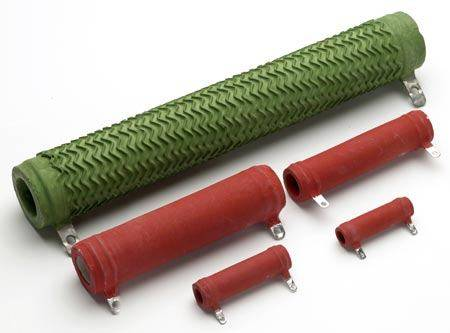# Simple circuit question

• I
pierce15
Let's say I have some wire with known constant resistivity and I make a coil out of it. Can this schematically be considered a resistor in series or parallel with an inductor, or neither? The voltage drop across the resistor would have to always be the same as across the resistor, which suggests parallel... Any thoughts?

Can this schematically be considered a resistor in series or parallel with an inductor, or neither?
It is a series connection. Power resistors used to be produced that way.

Here are some power wirewound resistors:•davenn
Gold Member
Let's say I have some wire with known constant resistivity and I make a coil out of it. Can this schematically be considered a resistor in series or parallel with an inductor, or neither?

there is only 1 resistive component (only 1 component, period), there isn't anything in parallel with it, other than the power source ( which doesn't count in this case)

the wire in a coil is still going to be a resistor as shown in the pic's that Svein has shown ... particularly the top one.
BUT because it is a coil it is going to have significant inductance

Dave

Gold Member
BUT because it is a coil it is going to have significant inductance
. . . . at a high enough operating frequency. No problem at 50HZ, though.
There is also a parasitic Capacitance (Parallel) that appears across the terminals. This will resonate with the nominal inductance and its effective inductance can be greater than nominal (approaching infinity at resonance). Thereafter, the component becomes capacitative. We're talking in terms of many tens of MHz or more (depending on the actual design of the Resistor. Resistors are often made with metal film with a spiral cut along the length of the cylinder (to trim the R value). That has a much lower inductance (only a very few turns) but the most expensive ones are trimmed during manufacture with a longitudinal groove (no spiral) and much better behaved at RF frequencies.
No component is ever exactly what's printed on the side!

•davenn
Gold Member
There is also a parasitic Capacitance (Parallel) that appears across the terminals. This will resonate with the nominal inductance and its effective inductance can be greater than nominal (approaching infinity at resonance). Thereafter, the component becomes capacitative. We're talking in terms of many tens of MHz or more (depending on the actual design of the Resistor.

yupUs ham radio guys would often used 50 Ohm wire wound resistors as higher power dummy loads and would have to use some parallel capacitance to negate the inductive effects so that the transmitter would see a load that looked "more' resistive than inductive.
This works great for the HF - VHF bands 1 - 150MHz. At 430 - 440MHz it became a little more touchy and we would often look for better
ways.

Dave

pierce15
Alright... So if we put an AC voltage across the circuit, it can be analyzed using an impedance of ## i\omega L + R ##, where L is the well known inductance of a coil?

Also, how would you find what the theoretical capacitance of this setup would be?